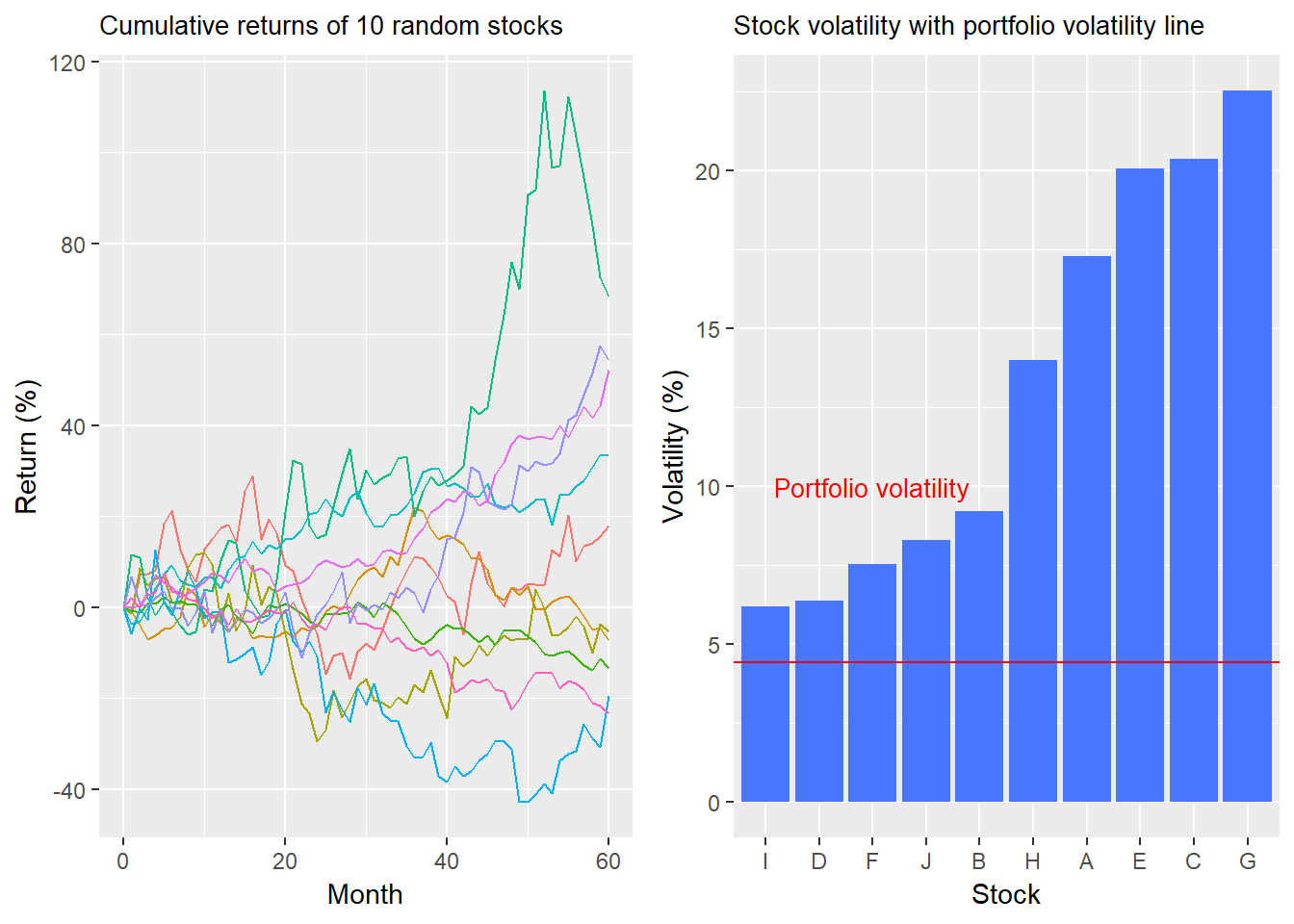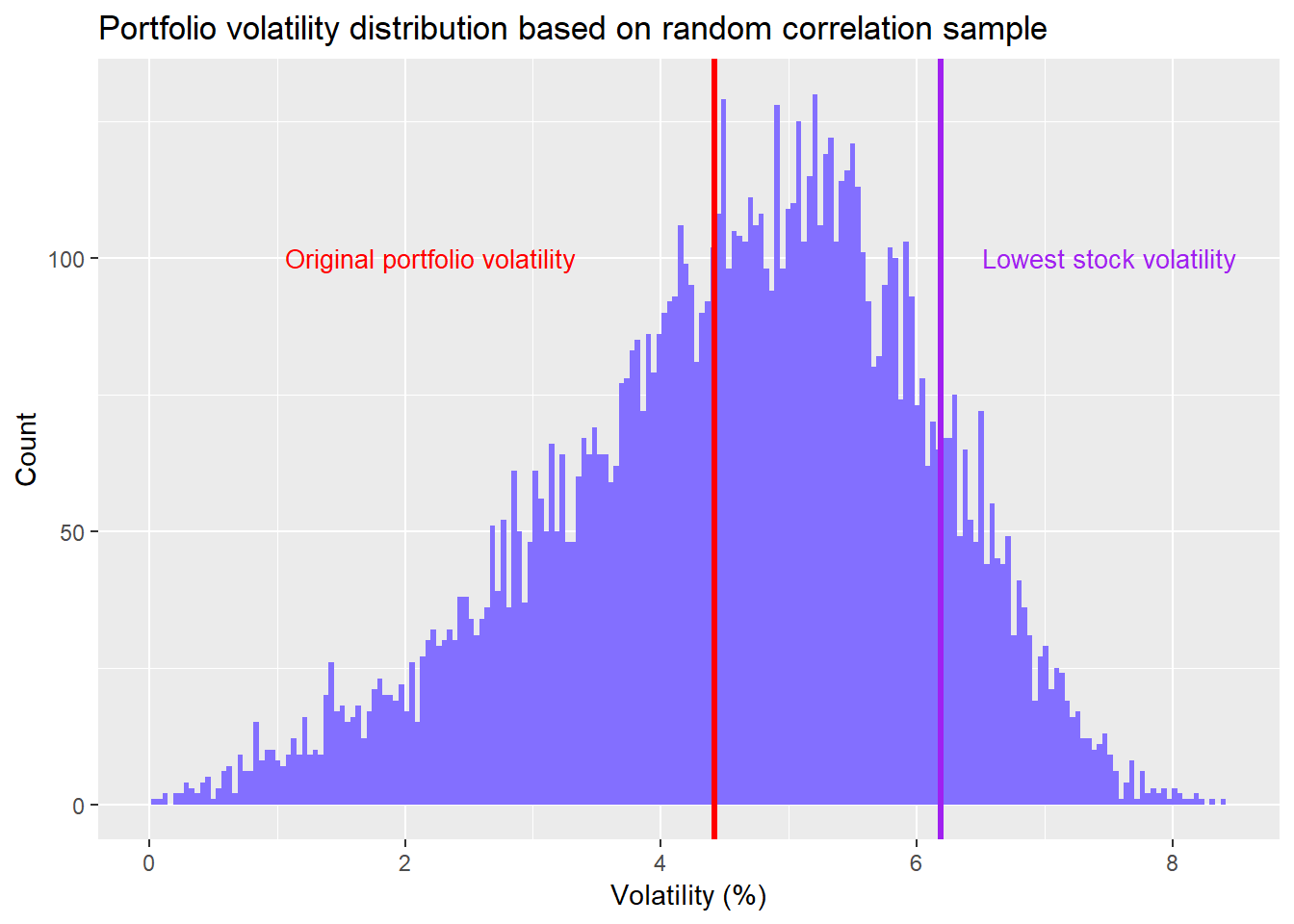In our last post, we took a detour into the wilds of correlation and returned with the following takeaways:

• Adding assets that are not perfectly positively correlated to an existing portfolio tends to lower overall risk in many cases.

• The decline in risk depends a lot on how correlated the stocks are in the existing portfolio as well as how the additional stocks correlate with all the existing assets.

• Correlation complicates how we answer our original question on whether an investor is always better off being diversified, rather than not. You can’t just look at simulations of random stock movements; you also need to simulate corrrelations too!

Seems like we’ve done a lot of work to arrive back where we started. Maybe even worse since it now appears like we know even more about what we don’t know. But don’t be discouraged. Recall that in our toy portfolio we found that diversification resulted in a better return-to-risk ratio 80% of the time. Also, if the portfolio was already not perfectly correlated, adding a less-than-perfectly correlated asset lowered volatility around 80% of the time.

Still, these are provisional conclusions for the following reasons:

• We only looked at one portfolio of ten stocks.
• We only used an equal weighting of those stocks.
• There was only one set of correlations among those stocks.

Let’s look at what happens when we create a random sample of correlations.

## Imagining bouncing balls

In our previous post, we likened trying to predict correlations to trying to imagine which way three balls would bounce when dropped from a second story window. And then trying to imagine how each of the bounces from each of the balls would relate to all of the bounces from all the other balls. Not easy.

But we can simulate a random selection of correlations using the power of R and aggregate the resulting risk calculations. We’ll let the computer imagine all the different balls bouncing in all their different ways. All we need to go is get a feel for the central tendency.

As a refresher, here’s our original return and volatility charts.Now we randomly simulate a set of correlations between the 10 stocks (equivalent to 45 pairs of corelations). This assumes that the stocks we generated have the same return and volatility profiles, but different correlations than what we originally created. We create 10,000 of these correlation matrices and from there compute the portfolio risk for each of these random sets of correlations.1 The equal-weighting of the stocks remains the same. We plot a histogram of the results below with the red line showing the original portfolio volatiliy and the purple line showing the stock with the lowest volatility.Here are the takeways. Even with random correlations, about 87% of the portfolios enjoy volatility that’s lower than even the least risky stock. However, about 59% of the portfolios are above the volatility of the original portfolio, supporting the notion that not only does correlation impact diversification, it is not always for the better. You can’t rely on one itereation to generalize the overall impact of diversificatiion.

Are we closer to answering our question on whether diversification is always better?

• A portfolio of stocks may lower overall volatility compared with owing any one stock. One point in favor of diversification.

• The reason why the (supposedly) diversified portfolio lowers risk (or does not) is due to correlation. Namely, not all stocks are strongly correlated, so they don’t all move in the same direction with the same velocity. But knowing correlation in advance is not easy. Neutral to diversification?

• Owning a bunch of stocks doesn’t mean you’ll be diversified. In this case, with the random correlations, most of the portfolios were less risky than the least risky stock. But about 13% of the time they weren’t. And we haven’t calculated risk-adjusted returns. Half a point against diversification?

We admit that the last point is a bit nit-picky. It’s hardly worth worrying about owning a portfolio that might be more risky than the least risky stock. Except in this case the least risky stock also had the highest risk-adjusted return! So 13% of the time you’d have been better off owning one stock rather than a portfolio of stocks. Seems like Warren Buffett’s rejection of diversification mentioned in our first post might hold water.

What if we changed the weightings? If we were to apply a thousand random weightings to a sample of a thousand random correlations, portfolio risk rises significantly. The percentage of portfolios that exceed the least risky stock increases to 27% from 13%. (Buffett’s starting to look even more right!) The percentage that exceed the original portfolio risk is now 68% vs. 59% previously. And this change is simply from altering the weightings on all ten stocks. What if we chose not to own some of those stocks? Needless to say, it would be tough to have a strong intuition on the potenital results except to guess that the range of outcomes could increase, to say nothing of what would hapen to the return-to-risk ratios.

But that is more than enough to digest for now. In our upcoming posts, we’ll look at risk-adjusted returns based on our random correlations and examine what happens to risk and risk-adjusted returns when we exclude some stocks from the portfolio. This should get us closer to the answering the diversification question.

Until then, here’s all the code behind the analysis and graphs:

```# Load package
library(tidyquant)
library(cowplot)

# Create toy portfolio
set.seed(123)
mu <- seq(-.03/12,.08/12,.001)
sigma <- seq(0.02, 0.065, .005)

mat <- matrix(nrow = 60, ncol = 10)
for(i in 1:ncol(mat)){
mu_samp <- sample(mu, 1, replace = FALSE)
sig_samp <- sample(sigma, 1, replace = FALSE)
mat[,i] <- rnorm(nrow(mat), mu_samp, sig_samp)
}

df <- as.data.frame(mat)
asset_names <- toupper(letters[1:10])
colnames(df) <- asset_names

# Cumulative returns
df_comp <- rbind(rep(1,10), cumprod(df+1))

# Return graph
ret_graph <- df_comp %>%
mutate(date = 0:60) %>%
gather(key, value, -date) %>%
ggplot(aes(date, (value-1)*100, color = key)) +
geom_line() +
labs(y = "Return (%)",
x = "Month",
title ="Cumulative returns for random stock sample") +
theme(legend.position = "none",
plot.title = element_text(size = 10))

# Create volatility date frame
vol <- df %>% summarise_all(., sd) %>% t() %>% as.numeric()
vol <- data.frame(asset = asset_names, vol = vol)

# Portfolio volatility
weights <- rep(0.1, 10)
port_vol <- sqrt(t(weights) %*% cov(df) %*% weights)
# round(port_vol*sqrt(12), 3)*100

# volatility graph
vol_graph <- vol %>%
mutate(vol = vol*sqrt(12)*100) %>%
ggplot(aes(reorder(asset,vol), vol)) +
geom_bar(stat = "identity", fill = "royalblue1") +
geom_hline(yintercept = port_vol*sqrt(12)*100, color = "red") +
labs(y = "Volatility (%)",
x = "",
title = "Stock volatility with portfolio volatility line") +
theme(legend.position = "none",
plot.title = element_text(size = 10)) +
annotate("text", x = 3,
y = 10,
label = "Portfolio volatility",
color = "red",
size = 3.5)

# Plot side by side graphs of returns and volatlities
plot_grid(ret_graph, vol_graph)

## Create random correlations
set.seed(123)
corr_list <- list()
combo <- expand.grid(vol\$vol, vol\$vol)
combo <- combo %>% mutate(Var3 = Var1 * Var2)
for(i in 1:10000){
test_mat <- matrix(nrow = 10, ncol = 10)
rand_cor <- runif(45, -1, 1)
test_mat[upper.tri(test_mat, diag = FALSE)] <- rand_cor
test_mat[lower.tri(test_mat, diag = FALSE)] <- rand_cor
test_mat[is.na(test_mat)] <- 1
cov_mat <- test_mat * combo\$Var3
corr_list[[i]] <- cov_mat
}

# Calculate portfolio volatility
rand_vol <- c()
for(i in 1:10000){
rand_vol[i] <- sqrt(t(weights) %*% corr_list[[i]] %*% weights)
}

# NaNs produced since some correlations produced randomly are impossible

# Create data frame and graph
rand_vol_df <- data.frame(vol = na.omit(rand_vol))

rand_vol_df %>%
ggplot(aes(vol*sqrt(12)*100)) +
geom_histogram(bins = 200, fill = "slateblue1") +
geom_vline(xintercept = port_vol*sqrt(12)*100, color = "red", lwd = 1.5) +
geom_vline(xintercept = min(vol\$vol) * sqrt(12) * 100, color = "purple", lwd = 1.2) +
# geom_vline(xintercept = mean_vol_port, lwd = 1.2) +
# geom_density(aes(y = .01*..count..), color = "blue") +
labs(x = "Volatility (%)", y = "Count",
title = "Portfolio volatility distribution based on random correlation sample") +
annotate("text", x = 2.2, y = 100,
label = "Original portfolio volatility",
color = "red", size = 3.5) +
annotate("text", x = 7.5, y = 100,
label = "Lowest stock volatility",
color = "purple", size = 3.5)

# occurence calculations
prob_above_port <- round(mean(rand_vol_df\$vol > as.numeric(port_vol)), 3)*100
prob_below_min <- round(mean(rand_vol_df\$vol < min(vol\$vol)),3) * 100
mean_vol_port <- round(mean(rand_vol_df\$vol)*sqrt(12), 3)*100
med_vol_port <- round(median(rand_vol_df\$vol)*sqrt(12), 3)*100
low_vol <- as.numeric(quantile(vol\$vol, probs = .2))
prob_above_low_vol <- round(mean(rand_vol_df\$vol > low_vol), 3)*100

# Run correlation samples with alternate weightings

# Create smaller sample of random correlations
set.seed(123)
sml_corr_list <- list()
for(i in 1:1000){
test_mat <- matrix(nrow = 10, ncol = 10)
rand_cor <- runif(45, -1, 1)
test_mat[upper.tri(test_mat, diag = FALSE)] <- rand_cor
test_mat[lower.tri(test_mat, diag = FALSE)] <- rand_cor
test_mat[is.na(test_mat)] <- 1
cov_mat <- test_mat * combo\$Var3
sml_corr_list[[i]] <- cov_mat
}

# Create alternative weights
set.seed(123)
port_wt <- matrix(nrow = 1000, ncol = 10)
for(i in 1:nrow(port_wt)){
a <- runif(10, 0, 1)
b <- a/sum(a)
port_wt[i,] <- b
}

# Create risk sample
rand_vol_1 <- c()
grid <- matrix(1:1000000, byrow = TRUE, ncol = 1000)
for(i in 1:1000){
cor_mat <- sml_corr_list[[i]]
for(j in 1:1000){
rand_vol_1[grid[i,j]] <- sqrt(t(port_wt[j,]) %*% cor_mat %*% port_wt[j,])
}
}

# Create data frame and graph
rand_vol_1_df <- data.frame(vol = na.omit(rand_vol_1))

# Occurrences
low_vol <- as.numeric(quantile(vol\$vol, probs = .2))
prob_above_low_vol_1 <- round(mean(rand_vol_1_df\$vol > low_vol), 2)*100
prob_above_port_1 <- round(mean(rand_vol_1_df\$vol > as.numeric(port_vol)), 2) *100
prob_below_min_1 <- round(mean(rand_vol_1_df\$vol < min(vol\$vol)),2) * 100```

1. For those matthematically inclined, we removed about 6-7% of the portfolios created because the random sampling produces correlations that are not possible.